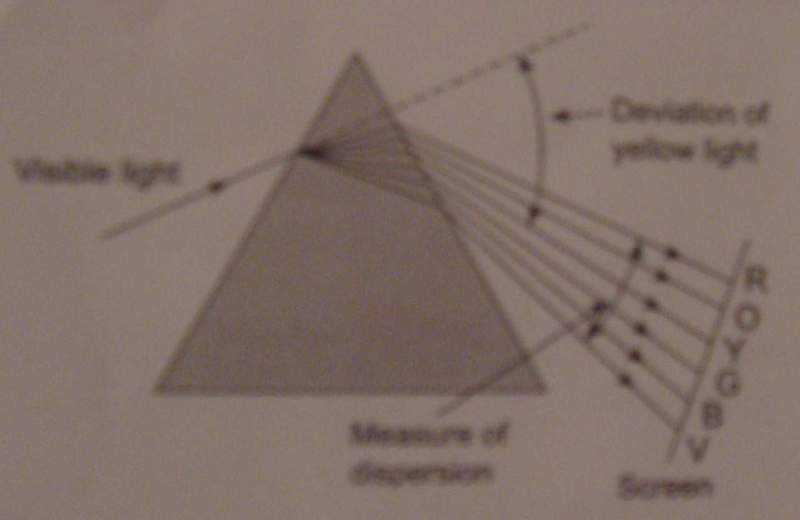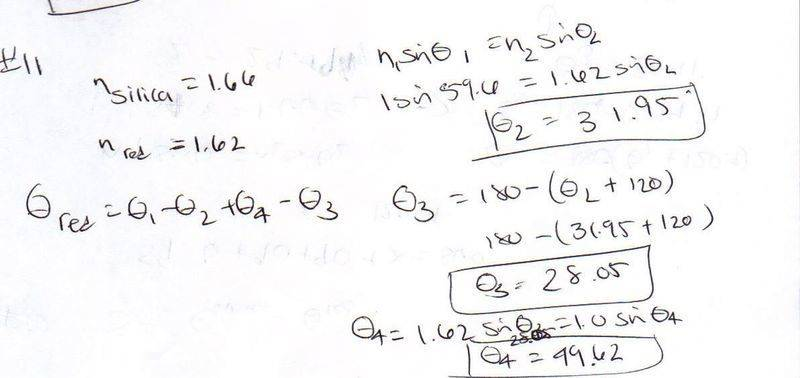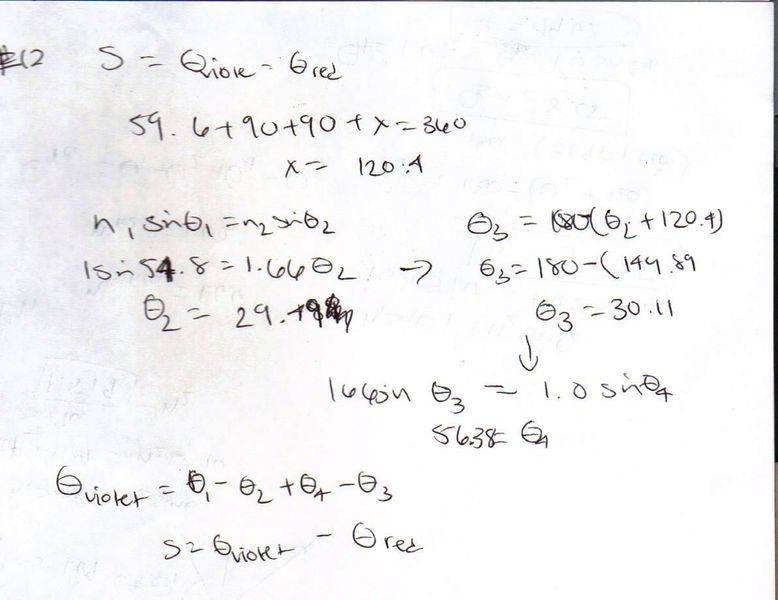# Angle of deviation and angle of incidence?

## Homework Statement

This is a two part problem:

11) The index of refraction for violet light in silica ﬂint glass is 1.66, and that for red light is 1.62.What is the angle of deviation for the red ray passing through a prism of apex angle 59.6◦ if the angle of incidence is 54.8◦? Answer in units of◦.

12) What is the angular dispersion of visible light with the same angle of incidence? Answer in units of◦.

## Homework Equations

n1$$\theta$$1=n2$$\theta$$2

## The Attempt at a Solution

My work:Can you tell me if I did it right? TIA. Sorry for the bad pics & handwriting!